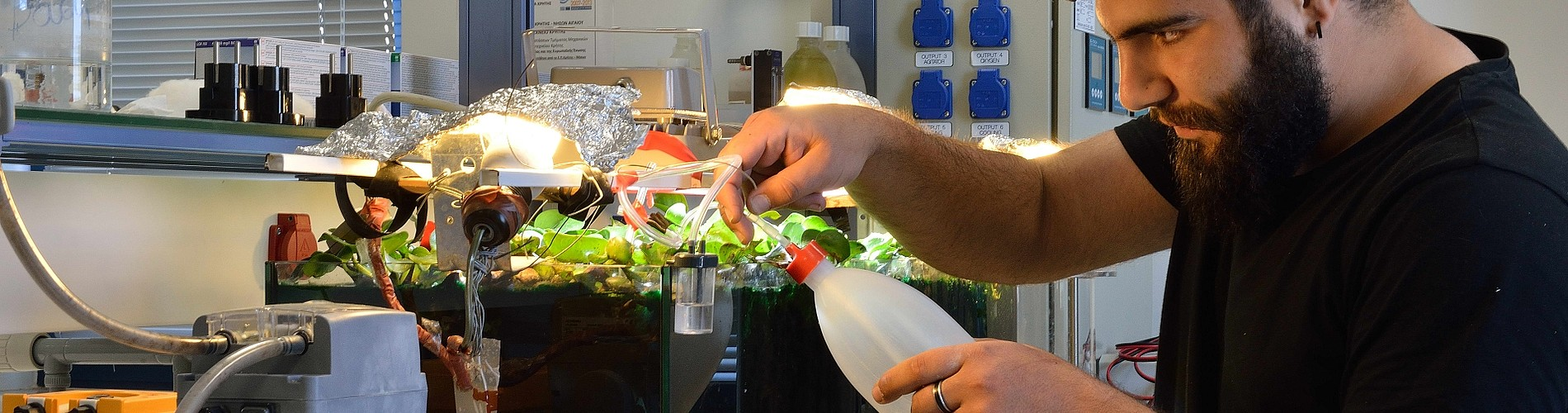# Applied Mathematics for Environmental Engineers

Course Information:

 Course ID: ΑΒ-308 Type of Course: Elective Semester: Fall ΕCTS: 9

Instructors: Associate Professor T. Daras, Associate Professor A. Manoussakis

(Α)   ORDINARY/PARTIAL DIFFERENTIAL EQUATIONS

• Y.A.Cengel, W.J.Palm III, Differential equations for engineers and scientists, McGraw Hill, 2013.
• W.E.Boyce – R.C.DiPrima, Elementary differential equations and boundary value problems, Wiley, 10th ed.

(Β)   SIGNALS –SYSTEMS – FOURIER TRANSFORMS

• Hwei Hsu, Signals and Systems, Schaums outlines, 2nd ed., 2011.

(C)   PROBABILITY – STATISTICS

• A.Papoulis, Probability, Random variables and Stochastic Processes, international ed, 4th, McGraw Hill, 2012.

(D)   TIME SERIES

• Chatfield,C. The analysis of time series. An introduction, Chapman & Hall.

Eclass:

• Registration required

Course objectives:

This is an introductory graduate course on the topics: ordinary and partial differential equations, signals and systems, Laplace and Fourier transforms, simple and multiple linear regression, cluster analysis, time series, knowledge necessary to an Environmental engineer. Using this knowledge he/she can solve, by constructing mathematical models, real life environmental problems. During the lectures, the analytical theory (in each one of the subjects) is given and mathematical models/scenarios for environmental problems are being constructed. SPSS, MINITAB, MATLAB software are also being introduced and implemented.

Syllabus:

 1st Week Ordinary differential equations. Initial value problems. O.D.E.’ s of first and second order. 2nd Week: Applications of O.D.E.’s to population models, exponential decay, mixing, law of cooling. Introduction to Partial Differential Equations. 3rd Week Laplace transform. Applications in mechanics, electricity e.t.c. 4th Week Signals and systems 5th Week Fourier transform of a signal.  Face recognition. 6th Week: Review of Probability. Conditional probability. Independence. Random variables 7th Week: Simple linear regression. SPSS software 8th Week: Multiple linear regression. Cluster analysis. 9th Week: Normality tests. Chi square test of independence. 10th Week: Introduction to time series (definition, characteristics, stationarity, autocorrelation function). MINITAB software 11th Week: Time series decomposition. Applications of time series. 12th Week: Smoothing methods.  Decomposition & smoothing comparison. 13th Week: Projects presentation

Assessment method:

• Homework problems (20%)
• Project (50%)
• Final exam (30%)

Last modification: 0-10-2019